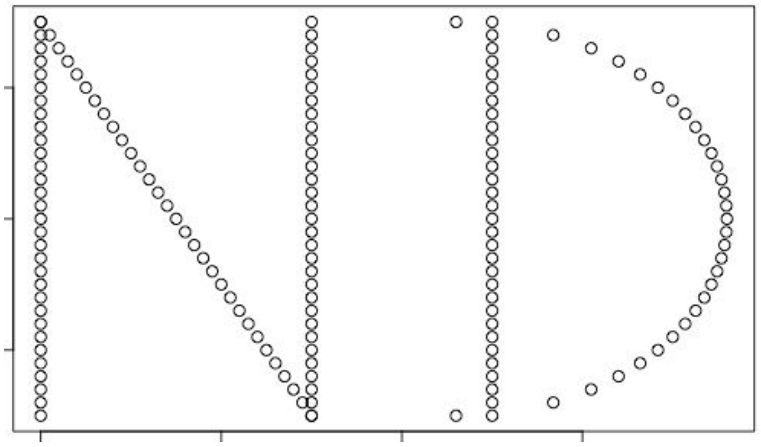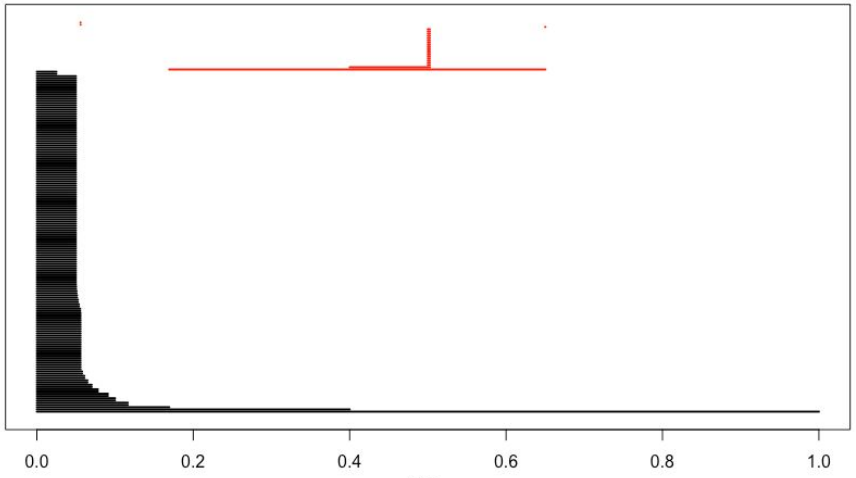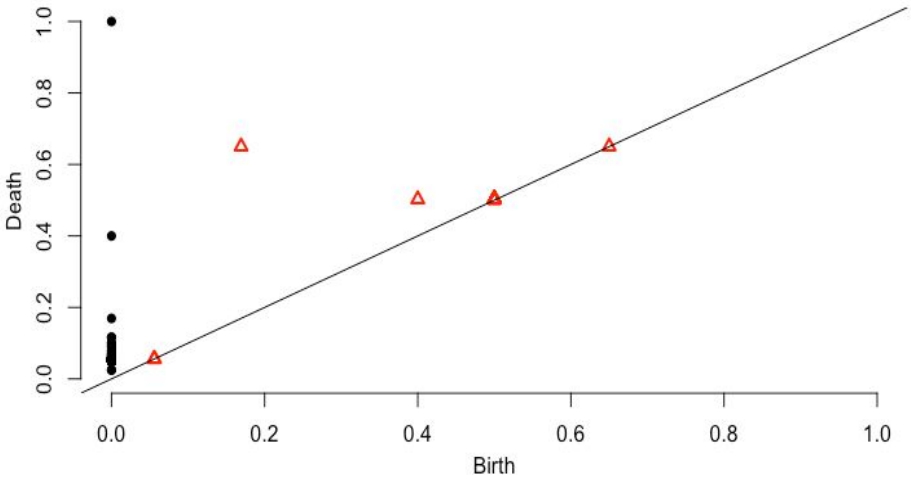# Persistent Homology Software: Demonstration of TDA

This post is part of a series.

An R package named TDA (Fasy et al. 2014) has persistent homology capabilities, which we will demonstrate on another ND logo dataset.


n_x <- c(rep(-1,31),0.005*seq(-200,-50,5),rep(-.25,31))
n_y <- c(0.01*seq(-75,75,5),-0.01*seq(-75,75,5),
0.01*seq(-75,75,5))

d_x <- c(rep(.25,31),
0.15+sqrt(0.75^2-(0.01*seq(-75,75,5))^2))
d_y <- c(0.01*seq(-75,75,5),0.01*seq(-75,75,5))

nd <- data.frame(x=c(n_x,d_x),y=c(n_y,d_y))
plot(nd)We’ll create a barcode diagram to display the dataset’s persistent homology in dimensions 0 and 1, for epsilon ranging from 0 to 1. The first homology components are colored black, while the second homology components are colored red.


Diag <- ripsDiag(X = nd, maxdimension = 2, maxscale = 1,
library = "GUDHI", printProgress = FALSE)
plot(Diag[["diagram"]], barcode = TRUE, main = "Barcode")In first homology we see one component that persists the whole way, capturing the N and D together, and another component that persists about halfway, capturing the separation between the N and the D. In second homology, we see one component that persists halfway and captures the hole in the D.

Birth-death diagrams are also used to display the same information as persistence barcodes:


plot(Diag[["diagram"]])There are also functions for calculating the Bottleneck and Wasserstein distances, which measure dissimilarity between homology diagrams. Below, we calculate these distances between the N and the D in the logo.


n <- data.frame(x = n_x, y = n_y)
d <- data.frame(x = d_x, y = d_y)

DiagN <- ripsDiag(X = n, maxdimension = 1, maxscale = 1)
DiagD <- ripsDiag(X = d, maxdimension = 1, maxscale = 1)

> print(bottleneck(Diag1 = DiagN[["diagram"]],
Diag2 = DiagD[["diagram"]], dimension = 1))
0.2404992

> print(wasserstein(Diag1 = DiagN[["diagram"]],
Diag2 = DiagD[["diagram"]], p = 2, dimension = 1))
0.05783988


References

1. Fasy, Brittany, Jisu Kim, Fabrizio Lecci, Clement Maria, and Vincent Rouvreau. "Introduction to the R Package TDA." CRAN. 2014.

This post is part of a series.